International
Tables for
Crystallography
Volume H
Powder diffraction
Edited by C. J. Gilmore, J. A. Kaduk and H. Schenk

International Tables for Crystallography (2018). Vol. H, ch. 3.9, pp. 353-356

## Section 3.9.5. Alternative methods for determination of calibration constants

I. C. Madsen,a* N. V. Y. Scarlett,a R. Kleebergb and K. Knorrc

aCSIRO Mineral Resources, Private Bag 10, Clayton South 3169, Victoria, Australia,bTU Bergakademie Freiberg, Institut für Mineralogie, Brennhausgasse 14, Freiberg, D-09596, Germany, and cBruker AXS GmbH, Oestliche Rheinbrückenstr. 49, 76187 Karlsruhe, Germany

### 3.9.5. Alternative methods for determination of calibration constants

| top | pdf |

#### 3.9.5.1. Standardless determination of the phase constant C

| top | pdf |

In order to determine the phase calibration constant C, it is common to obtain (i) a pure sample of the phase of interest that accurately reflects the form of the phase in the samples to be analysed, or (ii) a multiphase sample in which the phase concentration is known by other means (for example, chemical analysis or point counting). In some systems, there may be insufficient sample available to risk `contaminating' it with an internal standard, especially if the material needs to be analysed using other techniques. The addition of an internal standard may also introduce microabsorption problems or increase the complexity of patterns that are already highly overlapped. For other situations, the time frame demanded for the analysis may prohibit the time-consuming procedures of standard addition, data collection and separate determination of the phase calibration constant.

Zevin & Kimmel (1995) have described an approach to the derivation of phase constants which relies on having a suite of samples to be analysed that (i) have the same phases present in all samples and (ii) exhibit a wide range of composition of these phases in various samples in order to stabilize the analysis. If we reconsider the relationship between the weight fraction Wα and the observed intensity [equation (3.9.3)],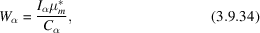and assume that all phases in the system are known and included in the analysis, we can introduce the additional constraint that the sum of all Wα's is unity (or at least a known value):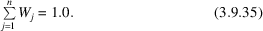In a system of n samples containing m phases, we can explicitly write the relationships expressed in equations (3.9.34)and (3.9.35)as a set of simultaneous equations: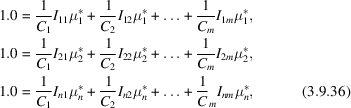where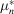is the mass absorption coefficient for the nth sample.

Knudsen (1981) has described a modification to this approach by including an internal standard in each of the samples to be analysed and using the ratio of intensities of the analyte and internal standard phases in place of the Inm in equation (3.9.36). While this eliminates the need to determine and use the mass absorption coefficient, the tedious procedure of adding and mixing an internal standard is required for each sample and for reasons described above may not be appropriate.

The relationships embodied in equations (3.9.36)can be expressed more simply in matrix notation as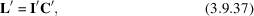where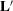is a column vector (dimensions 1 × n) containing the known (or assumed) sum of weight fractions for each sample (unity in this case),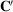is a column vector (dimensions 1 × m) containing the calibration constants for each phase and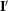is a rectangular matrix (dimensions n rows × m columns) containing the measured peak intensities (or scale factors) for each phase multiplied by the sample mass absorption coefficient.

A least-squares solution of equation (3.9.37)to derive the value for C for each phase can be calculated using matrix-manipulation methods (Knudsen, 1981):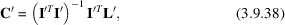where the superscripts T and −1 represent the transpose and inverse matrix functions, respectively.

Accuracy in the calculation of the individual values of C is improved by having (i) phases of the same or similar composition in all samples and (ii) a wide range of concentrations of each phase across the sample suite. These conditions may be met in, for example, mineral exploration samples where a limited number of phases are present in a drill core but their abundance varies as a function of depth. In mineral processing or industrially based material manufacture, the goal is usually to control the system to minimize compositional variation in the product. The side effect of this is that the values of intensity in matrix I have too little variation, resulting in large errors in the derived values for C. In the limiting case, the system may become indeterminate with no unique solution available. To overcome this, forced or accidental changes to processing conditions may introduce sufficient compositional variation to stabilize the determination of the C values through equation (3.9.38). Alternatively, physical or chemical separation of selected components may be sufficient to provide the required compositional variation. Knudsen (1981) provides a detailed statistical analysis used in the determination of the errors in the phase constants.

While Zevin (Zevin & Kimmel, 1995) and Knudsen (1981) have demonstrated the application of this approach for single-peak methods, it is equally applicable if scale factors derived from whole-pattern fitting or Rietveld-based methods are used.

#### 3.9.5.2. Demonstration of the Zevin approach

| top | pdf |

The sample 1 suite from the IUCr CPD round robin on QPA again provides an ideal platform for demonstrating the applicability of this method due to the wide variation of concentration of the constituent phases. A measure of intensity was derived using an hkl_phase (see Section 3.9.6) in which the peak positions were constrained to the space group and unit-cell parameters but the individual peak intensities were refined to empirical values using a pure sub-sample of each of the three phases. For the analysis of the samples, the relative peak intensities were fixed and an overall scale factor S for each phase in each sample (eight samples, three replicates, three phases), multiplied by the mass absorption coefficient calculated from the XRF-determined composition, was used as the measure of intensity. These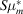values then formed the intensity matrix I in equations (3.9.37)and (3.9.38)while all values in the vector L were assumed to be 1.0 (i.e. all samples were assumed to be fully crystalline). Microsoft Excel provides a useful platform for these calculations since it contains all of the matrix-manipulation functions required by equation (3.9.38). The determined values for C for the three phases are given in Table 3.9.3. The values in the C/Ccorundum column should be compared with the values derived in Section 3.9.4.3above.

 Table 3.9.3| top | pdf | Phase calibration constants for corundum, fluorite and zincite determined using the Zevin (Zevin & Kimmel, 1995) and Knudsen (Knudsen, 1981) method
 The RIR values were derived earlier in this chapter.
PhaseCC/CcorundumRIR
Corundum 240.91 1.0 1.0
Fluorite 874.27 3.629 3.617
Zincite 1190.81 4.943 4.856

Application of these C values to the analysis of all samples via equation (3.9.34)yields the results given in Fig. 3.9.9. The results, displayed as bias from the known values, show that at all concentration ranges the analyses are within about ±1% of the weighed values. The important point to note here is that there has been no prior calibration conducted to obtain this result; the system is self-calibrating and has only relied on having a wide range of concentrations of the three phases in the sample suite. The only prior knowledge used in the analysis is (i) a measure of peak intensity embodied in the empirical phase scale factor and (ii) an estimate of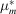for each sample calculated from the elemental composition.Figure 3.9.9 | top | pdf |Plot of the bias (known − determined) in the analysed phase abundances using the Zevin & Kimmel (1995) approach for corundum (black diamonds), fluorite (open triangles) and zincite (crosses). The 72 determinations derive from three replicates of eight mixtures containing three phases each.

#### 3.9.5.3. Experiment constant – a whole-sample approach

| top | pdf |

Earlier discussion has noted that the experiment constant K used in equation (3.9.21)can be determined using (i) a standard pure phase or mixture measured separately from the measurement of the actual unknown mixture being analysed, or (ii) using a phase that is present in the sample in a known amount. However, in some cases, these approaches are not always effective in producing reliable values of K because the methodology assumes that the mass of sample contributing to the diffraction process is constant. While this condition is true for infinitely thick samples in Bragg–Brentano geometry, it is unlikely to be true for capillary or flat-plate samples in transmission geometry. In these cases, the sample thickness and packing density will have a significant influence on the amount of sample contributing to the diffraction process and hence on the observed intensity and the derived values of K. Therefore, a K value determined from one capillary sample is unlikely to be applicable to another capillary even though all other instrumental conditions remain the same. However, for in situ studies, a K value determined at the start of an experiment should remain valid as the analysis proceeds.

K can also be determined using the whole sample, rather than an individual phase. Since the determined value of K then applies equally to all phases in the sample, equation (3.9.21)can be summed over all analysed components thus: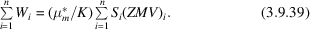If the crystallinity of the sample is known (or can safely be assumed), then individual phase abundances are not required and K can be calculated from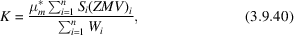where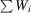is the assumed crystallinity of the entire sample.

For a sample that is 100% crystalline and all components included in the analysis, then the denominator is unity and K is simply the sum of the product of the scale factors and their respective ZMV's multiplied by the mass absorption coefficient of the entire sample.

For in situ studies where a reaction or process is examined dynamically, sealed capillary sample geometry is frequently used. In this environment, the chemical composition of the capillary contents will not change during the course of the reaction even though individual phases may be undergoing transformation. Equation (3.9.40)can be further simplified since the overall sample mass absorption coefficient remains constant throughout the reaction and can therefore be deleted and its effect incorporated into K.

This whole-sample approach to the determination of K is also useful in systems where there are residual errors that may not be evident when equation (3.9.21)is used with the concentration of a single phase. By way of demonstration, the sample 1 suite from the IUCr CPD round robin on QPA has been used to calculate K in two distinct ways:

 (1) Phase specific: Three replicate measurements of the eight mixtures were analysed using a Rietveld-based surface-analysis approach (Stinton & Evans, 2007). This approach refines a single model to all data sets in the suite simultaneously allowing parameters that are common to all samples to be determined with a greater degree of certainty. Since the sample suite contains corundum, fluorite and zincite in a wide range of concentrations, application of a rearranged equation (3.9.21)using the refined scale factors results in 72 separate determinations of K. The value offor each sample was derived from XRF-determined compositions using equation (3.9.5), while the values for Wi were taken from the known weight additions. (2) Whole sample: Equation (3.9.40)was applied to each of the 24 data sets (i.e. three replicates each of the eight samples) assuming (i) that all phases were known and fully crystalline, i.e.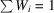; and (ii)for each sample was derived in the manner described above.

Fig. 3.9.10shows the 72 individual determinations of K from the phase-specific method as a function of known phase concentration. At high concentrations, the values for K derived from each of the three phases are similar indicating that, for effectively pure phase samples, the approach embodied in equation (3.9.21)is valid. However, if K is determined using the known concentration of a single phase at a lower concentration in a multiphase sample, then residual errors in the measurement of pattern intensity serve to reduce its accuracy. At lower concentrations of corundum, there is a systematic increase in the determined value of K resulting from a small microabsorption effect present in these samples. Since corundum has the lowest mass absorption coefficient of the three phases in this system its intensity, and hence Rietveld scale factor, is slightly overestimated relative to the fluorite and zincite. This results in an overestimation of the value of K relative to an ideal sample; the magnitude of this difference is about 5% relative. Use of these values for subsequent analysis will result in an underestimation of phase concentrations using equation (3.9.21). The converse is true if fluorite or zincite is used to determine K.Figure 3.9.10 | top | pdf |Plot of the experiment constant K as a function of known phase concentration for corundum (closed diamonds), fluorite (open triangles) and zincite (crosses) using the phase-specific method. The 72 determinations derive from three replicates of eight mixtures containing each of the three phases. The solid line is the mean of 24 values determined using the whole-sample approach. The dashed lines represent ±2 standard deviations about this mean.

However, if the whole-sample approach embodied in equation (3.9.40)is used for the determination of K, these residual sample-related aberrations can be eliminated; the results of the determination of 24 values of K using this approach are also included in Fig. 3.9.10. The mean of all 24 determinations is 427.6 (3.7) representing a relative error of <0.8%. The important point to note here is that knowledge of the individual phase concentrations is not needed; the only assumption needed relates to the total crystallinity of each sample.

For in situ studies, using equation (3.9.40)to calculate K at each step i in the reaction (defined as Ki) can be useful in deriving details of the reaction mechanism. If Ki increases as the reaction progresses, this may be indicative of increasing crystallinity in the sample. Reductions in Ki during the reaction may point to the formation of intermediate amorphous material or unidentified crystalline components, the total concentration of which can be readily calculated using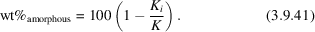Application of this can be demonstrated using sample 3 from the QPA round robin (Scarlett et al., 2002), as it contains the same three crystalline phases as the sample 1 suite with the addition of 29.47 wt% amorphous silica flour. Calculation of Ki for sample 3, based only on the three crystalline phases, results in a value of 301.8. Substituting this into equation (3.9.41)along with the previously determined value of K (427.6) gives a measured amorphous content of 29.42 wt% – this is in good agreement with the known weighed amount. The important point to note here is that the data for sample 3 were collected at the same time, and under the same instrumental conditions, as for sample 1, which ensured that the true value of K was the same for all data.

### References

Knudsen, T. (1981). Quantitative X-ray diffraction analysis with qualitative control of calibration samples. X-ray Spectrom. 10, 54–56.Google Scholar
Scarlett, N. V. Y., Madsen, I. C., Cranswick, L. M. D., Lwin, T., Groleau, E., Stephenson, G., Aylmore, M. & Agron-Olshina, N. (2002). Outcomes of the International Union of Crystallography Commission on Powder Diffraction Round Robin on Quantitative Phase Analysis: samples 2, 3, 4, synthetic bauxite, natural granodiorite and pharmaceuticals. J. Appl. Cryst. 35, 383–400.Google Scholar
Stinton, G. W. & Evans, J. S. O. (2007). Parametric Rietveld refinement. J. Appl. Cryst. 40, 87–95.Google Scholar
Zevin, L. S. & Kimmel, G. (1995). Quantitative X-ray Diffractometry. Springer-Verlag New York, Inc.Google Scholar